Courses

# Solved Examples : Surface Chemistry (IIT-JEE & AIPMT) Class 12 Notes | EduRev

## Chemistry Class 12

Created by: Mohit Rajpoot

## Class 12 : Solved Examples : Surface Chemistry (IIT-JEE & AIPMT) Class 12 Notes | EduRev

The document Solved Examples : Surface Chemistry (IIT-JEE & AIPMT) Class 12 Notes | EduRev is a part of the Class 12 Course Chemistry Class 12.
All you need of Class 12 at this link: Class 12

Ex.1 The charge on the colloidal particle of soap in its solution developed -

(A) By preferential adsorption of ions

(B) The effective ion of soap micelle carries negative charge

(C) The effective ion of soap micelle carries positive charge

(D) None of the above

Sol. B

RCOONa  →  RCOO¯ + Na+

The active species of soap is negatively charged.

Ex.2 In order to coagulate the impurities present in water. Which is the most effective ion present in the `alum' -

(A) K

(B) SO42-

(C) Al

(D) None of these

Sol. C

The impurities carry negative charge hence Al will exhibit maximum coagulating power.

Ex.3 Which is not the property of the hydrophilic sols-

(A) High concentration of dispersed phase can be easily attained

(B) Coagulation is reversible

(C) The charge of the particle depends upon the pH values of the dispersion medium. It may be positive or negative

(D) Viscosity and that surface tension are about the same as of dispersion medium.

Sol. D

Viscosity of hydrophilic sol is much higher than dispersion medium while surface tension of the sol is much lesser than water. This is attributed to the higher concentration of the sol itself.

Ex.4 If water is chosen as dispersion medium, then the sol formed will be called -

(A) Alcohosol

(B) Benzosol

(C) Hydrosol

(D) Aerosol

Sol. C

If water is chosen as dispersion medium then the colloidal system will be called hydrosol.

Ex.5 The number of moles of lead nitrate needed to coagulate 2 mole of colloidal [AgI]I- is-

(A) 2

(B) 1

(C) 1/2

(D) 2/3

Sol. 2[Ag I] I- + Pb2+  → PbI2  + 2AgI

Thus, one mole of Pb(NO3)2 is required to coagulate 2 mole of [AgI] I-.

Ex.6 Maximum hydrophobic character will be shown by

(A) Glycine

(B) Stearic acid

(C) Glucose

Sol. D

Ex.7 Fe(OH)3 sol obtained by peptization when subjected to `electrophoretic effect' -

(A) Colloidal particles will migrate towards anode

(B) Colloidal particles will migrate towards cathode

(C) Colloidal particles remain stationary

(D) Colloidal particles migrate towards both the electrode

Sol. B

Ferric hydroxide is a positive sol Fe(OH)3/Fe3+ , it will migrate towards cathode.

Ex.8 Which of the following solution is positively charged -

(A) As2S3

(B) Fe(OH)3

(C) Au

(D) Starch

Sol. B

Fe(OH)3/Fe3+  is a positively sol particle.

Ex.9 Dispersion of AgCl in water is called -

(A) Hydrophilic sol

(B) Emulsion

(C) Benzosol

(D)Hydrophobic colloid

Sol. D

Colloidal system AgCl is called, hydrophobic colloid.

Ex.10 The stability of lyophilic colloid is due to -

(A) Charge on the solution particles

(B) Layer of dispersion medium over the solution particles

(C) Smaller size of solution particles

(D) Bigger size of solution particles

Sol. B

Lyophilic colloids are of bigger size and this accounts for their stability.

Ex.11 The volume of nitrogen gas at 0ºC and 1.013 bar required to cover a sample of silica gel with unimolecular layer is 129 cm3 g-1 of gel. Calculate the surface area per gram of the gel if each nitrogen molecule occupies 16.2 × 10-20 m2.

Sol. 568 m2 g-1

PV = nRT

1.013 × 0.129 = n × 0.0821 × 273

n = 0.00583 mol

Area occupied

= 0.00583 × 6.023 × 1023 × 16.2 × 10-20

Ex.12 The rate of decomposition of acetaldehyde into methane and CO in the presence of I2 at 800 K follows the rate law

Rate = k[CH3CHO][I2]

The decomposition is believed to go by the two steps mechanism

CH3CHO + I2  → CH3I + HI + CO

CH3I + HI  → CH + I2

What is the catalyst for the reaction ? Which of the two steps is a slower one ?

Sol. I2 is catalyst ; first step is slow.

Ex.13 A catalyst lowered the activation energy by 25 kJ/mol at 25ºC. By how many times will the rate slow ?

Sol. 24069 times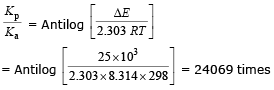Ex.14 Discuss the effect of temperature on the degree of adsorption of N2 on the surface of iron.

Sol. At room temperature, practically there is no adsorption of N2 gas on the surface of iron. At 83 K, nitrogen is physically adsorbed on the surface of iron. Its degree of adsorption decreases with rise in temperature and becomes almost nil at room temperature. At 773 K and above, there is chemical adsorption of nitrogen on the surface of iron.

Ex.15 One gram of charcoal adsorbs 100 mL of 0.5 M CH3COOH to form a mono-layer and thereby the molarity of acetic acid is reduced to 0.49 M. Calculate the surface area of the charcoal adsorbed by each molecule of acetic acid. Surface area of charcoal = 3.01 × 102 m2/gm.

Sol. Number of moles of acetic acid initially present =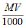=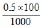= 0.05

Number of moles of acetic acid left =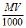=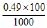= 0.049

Number of moles of acetic acid adsorbed = 0.05 - 0.049 = 0.01 mol

Number of molecules of acid adsorbed = 0.001 × 6.023 × 1023 = 6.023 × 1020

Area occupied by single molecule of acetic acid =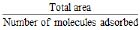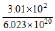= 5 × 10-19 m2

Ex.16 1.30 cm3 of N2 gas at STP is adsorbed per gram of silica gel. The area occupied by nitrogen molecule is 0.16 nm2. What is the surface area per gram of silica gel ? (NA = 6.023 × 1023)

(A) 1.6 m2 g-1

(B) 5.568 m2 g-1

(C) 3.48 m2 g-1

(D) 4.42 m2 g-1

Sol. B

Number of N2 molecules adsorbed per gram of silica gel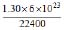= 3.48 × 1019

Area of cross-section of molecule = 0.16 nm2

= 1.6 × 10_19 m2

Surface area covered per gram of silica gel

= 1.6 × 10_19 × 3.48 × 1019 = 5.568 m2

Ex.17 Plot of log(x/m) against log P is a straight line inclined at an angle of 45º. When the pressure is 0.5 atm and Freundlich parameter, k is 10, the amount of solute adsorbed per gram of adsorbent will be : (log 5 = 0.6990)

(A) 1 g

(B) 2g

(C) 3 g

(D) 5 g

Sol. D

log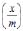= log k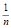log P= tan 45º = 1

log k = log 10 = 1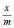= k(P)1/n = 10(0.5)1 = 5

When m = 1 g, x = 5 g

Ex.18 In homogeneous catalytic reactions, there are three alternative paths AB and C (shown in the figure). Which one of the following indicates the relative case with which the reaction can take place ?

(A) ABC

(B) CBA

(C) BCA

(D) ABC

Sol. Activation energy in the different paths lies in the following sequence : CB <A.

Lesser is the activation energy, greater is the ease with which the reaction can take place.

CBA (Deceasing case with which the reaction can take place.)

Ex.19 The coagulation of 100 mL of a colloidal solution of gold is completely prevented by adding 0.25 g of starch to it before adding 10 mL of 10% NaCl solution. Find out the gold number of starch.

Sol. 10 mL of 10% NaCl solution is added to 100 mL of solution of gold.

Thus, 1 mL of 10% NaCl solution has been added to 10 mL solution of gold.

Since, 100 mL gold solution required = 0.25 g starch

= 0.25 × 103 mg starch

So, 10 mL gold solution required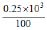× 10

= 25 mg starch

Thus, by definition, the gold number of starch is 25.

Ex.20 The coagulation of 100 mL of colloidal solution of gold is completely prevented by addition of 0.25 g of a substance "X" to it before addition of 1 mL of 10 % NaCl solution. The gold number of "X" is-

(A) 0.25

(B) 25

(C) 250

(D) 2.5

Sol. Number of milligrams of protective colloid added in 10 mL of colloidal gold to prevent its coagulation on addition of 1 mL of 10% NaCl solution is called its gold number.

Gold number of present colloid = 25

Discussion Questions

(1) How will you differentiate between a true solution and a colloidal dispersion?

(2) Identify some sols (colloids) that you use in your daily life and mention their importance.

(3) How do colloids acquire a charge? Why is ferric hydroxide/aluminium hydroxide sol prepared in the experiment, positively charged while arsenious sulphide sol is negatively charged?

(4) What is coagulation? How is coagulation different from peptization?

(5) How can you convert a colloidal dispersion of sulphur into a true solution?

(6) Out of lyophilic and lyophobic sols, which one can be easily converted into a gel and why?

(7) Differentiate between a gel and a sol.

(8) What are the applications of colloids in the field of Medicine, Defense and in Rocket Technology?

(9) How can you make the process of dialysis quick? What are the limitations of this technique?

(10) Name a reagent other than soap, which can be used as an emulsifying agent in the oil in water type emulsion.

(11) Milk is said to be a stable emulsion. What provides stability to milk?

(12) Can two miscible liquids form an emulsion?

(13) Why do separation of layers of different oils forming an emulsion with water take different time?

(14) What are the points of similarity and dissimilarity among sol, gel and emulsion?

(15) Suggest a test to distinguish between Oil in Water and Water in Oil type of emulsions.

(16) Give some examples of emulsions that you come across in daily life.

(17) Dettol forms an emulsion in water. How does this emulsion get stabilised?

Offer running on EduRev: Apply code STAYHOME200 to get INR 200 off on our premium plan EduRev Infinity!

,

,

,

,

,

,

,

,

,

,

,

,

,

,

,

,

,

,

,

,

,

;